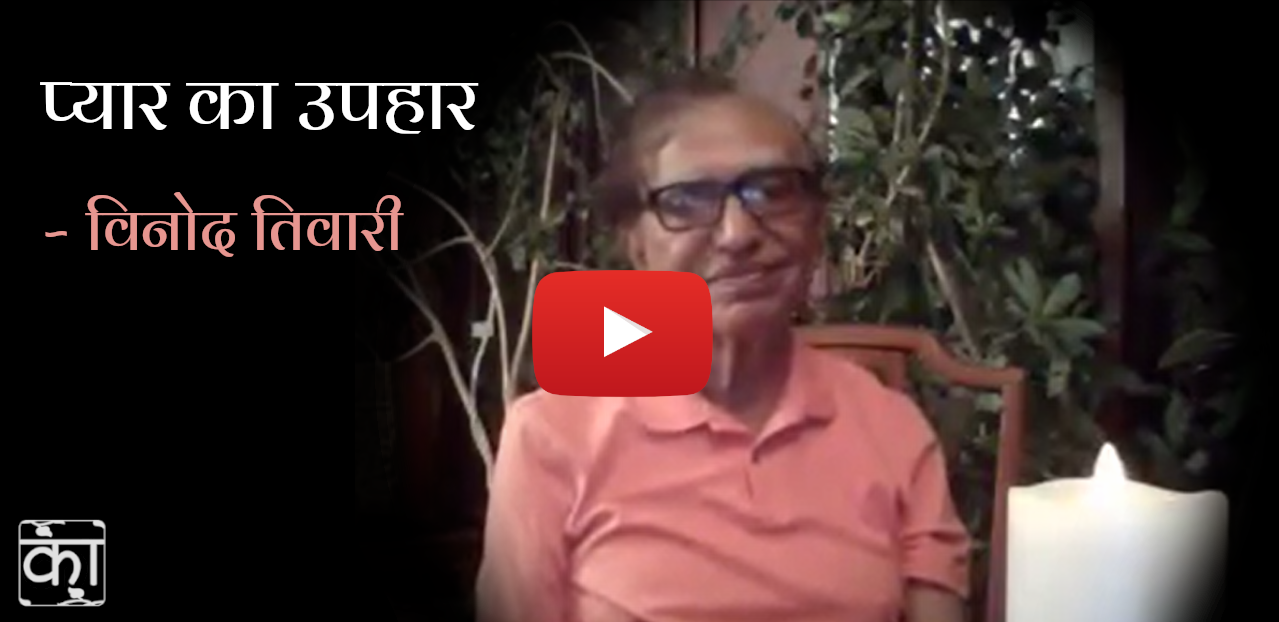﻿ Part 2. Maatraa in Metered (Chhandobaddh) Poems. Basic Structure of Hindi Poetry. Kaavyaalaya: The House of Hindi Poetry

Basic Structure of Hindi Poetry
Part 2 - Maatraa in Metered (Chhandobaddh) Poems

Vinod Tewary and Vani Murarka
(Editors, Kaavyaalaya.org)
Email address: [email protected]

Preface
This is Part 2 of the article series on the basic structure of Hindi poetry. The whole article is in 4 parts as listed below:
Part 1: Structural units of a poem.
Part 2: (This part) Maatraa (मात्रा) or Meter of a Poem for Tukaant (तुकान्त), Chhanddobaddh (छन्दोबद्ध) Poetry - i.e. poems that follow a clear rhyme and rhythm. These are perhaps the most important ingredients of the craft of poetry.
Part 2a: Software “Geet Gatiroop” to help in Maatraa counting
Part 3: Rhythm in Mukt-Kavita (free verse) based on Maatraa and other structures.
Part 3: Maatraa or the meter of poems – for Atukaant (अतुकान्त) poetry or Mukt Kavita (मुक्त कविता), i.e. free verse.
Part 3a: Geet Gatiroop software for Mukt-Kavita
Part 4: Correspondence with Urdu poetry - Although this article mainly deals with Hindi poetry, some description of Urdu poetry is included because of the close inter-relationship between the two.

1. Introduction
In this part, we are going to discuss maatraas in the context of the structure of Hindi poems continuing from Part 1. The Maatraa counting system will be described with examples from तुकान्त and छंदोबद्ध Hindi poems and some Urdu ghazals. These are the poems that follow a clear pattern of rhyme and rhythm. In Part 3, the maatraa structure of Mukta Kavita will be discussed. A little bit of maatraa count in Indian music is also included in Part 3 in order to show the correspondence between poetry and music.

Maatraa (मात्रा) is an important aspect of the craft of poetry and presumably applies to all languages. It defines the meter of the poem. In English maatraas are counted in terms of syllables. In Hindi, inherited by Sanskrit, the rules are very specific and precise. The maatraa part of Urdu ghazal is simlar though urdu ghazal has its own rules. This text is based upon our understanding which is admittedly elementary. For an authentic and more detailed description, consulting a proper book on "काव्य शास्त्र" is recommended.

2. Maatraa definition and counting system
Each letter in a poem contributes a maatraa that can be 1, or 2, depending upon whether it has a short or long pronunciation. Half letters contribute 0 or 1 depending upon whether they are pronounced separately or get telescoped into the following letter. Maatraas in poetry are analogous to the maatraas in Indian music as interpreted by tabla.

Maatraa in Hindi/Urdu is defined using the following system: the short swar (vowels) अ, इ, उ, are 1 maatraa, and the long swar आ, ई, ऊ,ऐ and औ are 2 maatraa. The swar ए and ओ can have 1 or 2 maatraas depending upon how they are pronounced.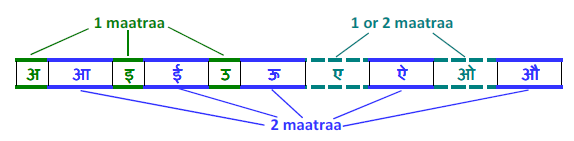This flexibility of 1 or 2 maatraas for the swar ए and ओ based on pronunciation exists more in Avadhee (अवधी) and Urdu (उर्दू). Examples are given below demonstrating this. However, in proper Khadee Bolee (खड़ी बोली) and its pronunciation, both ए and ओ consume 2 maatraas each. This is clearly followed in the poetry of the stalwarts of Khadee Bolee Hindi poetry in such as Dinkar, Bachchan, Subhadra Kumari Chauhaan etc. Nowadays due to the influence of Urdu and the movies the use of 1 maatraa for ए and ओ is found even in Khadee Bolee Hindi poetry. For Khadee Bolee, this is somewhat of a compromise. In Urdu, this is perfectly valid.

Half letters at the beginning of a word usually do not contribute to the maatraa count, because their pronunciation is telescoped into the following word. For example the half k in klesh (क्लेश) or half p in pyaar has 0 maatraa because 'k' is pronounced jointly with 'l' in 'klesh' and 'p' is pronounced jointly with 'yaa' in pyaar. When a half letter occurs inside a word then it contributes 1 maatraa like 'sh' in 'kasht' (कष्ट) or 'l' in 'kalp' (कल्प).

In Hindi poetry there is a line called sthaayee (स्थायी) and then a piece (like a stanza or chhand) called अंतरा as described in Part 1. There are different rules for different styles of Hindi poetry. The main rule is that the two lines that are supposed to rhyme must have the same total number of maatraas plus the same meter, that is the structure of maatraas (order of maatraas). If the first line starts with a maatraa structure as 1+2, then the next line must have the same structure 1+2. It is important to note that 1+2 cannot match with 2+1. However 2 can match with 1+1 so 1+2 or 2+1 can both match 1+1+1. For example " राह " (2+1) will not match with " रहा " (1+2) but both will match with " सड़क ".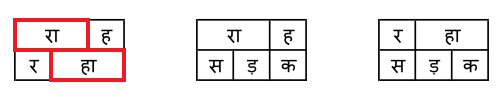Rules of maatraa in poetry follow exactly the same pattern as the rhythm in music. The maatraa in poetry has the same meaning as the maatraa in tablaa. This gives some liberty in the maatraa count. In music, one can elongate a swar or combine two swars into one either by merging the two swars or dragging a swar into another one - the so called ghaseet in Indian music (a rather unromantic word). In music, you would often see a singer pronouncing a long 'आ' or 'ई' as short 'अ' or 'इ'. This reduces the maatraa from 2 to 1. It is managed in music through tabla. In poetry, it is a compromise. In music one can also have half maatraa (for reducing the maatraa count to maintain the rhythm) which is not recommended in poetry though it is not uncommon.

3. Examples- Chhandobaddh poems- Hindi
Now let us take some examples. First take the perfect example of a chaupaayee from Tulsidas. For him writing poetry was a poojaa so he did not make compromises in the structure of his poetry. Consider the following from रामचरितमानस. The maatraa count following the above rule is given below each line grouped separately in parenthesis for each word:

 उमा(1+2) कहउं(1+1+1) मैं(2) अनुभव(1+1+1+1) अपना(1+1+2) = total 16 सत(1+1) हरि(1+1) भजन(1+1+1) जगत(1+1+1) सब(1+1) सपना(1+1+2) = total 16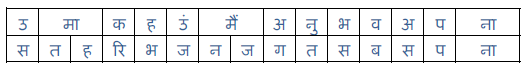Note that each line has a total of 16 maatraas and match with each other. The more important thing is the distribution of maatraas which is identical in both the lines. The maatraas of individual words do not have to match. Just the series has to match. If a maatraa 2 occurs in the upper line, the maatraas at the corresponding position in the lower line are either 2 or 1+1. As explained in the previous para, 2 matches with 1+1 or 2 and vice versa. In fact the maatraa structure of the entire line does not have to be exactly identical in the middle of the line. The most important thing is they need to match at the beginning and the end of the line that are the ascent and the descent of the line. The maatraa distribution of each line and the periodicity defines the laya of the poem. In the language of tabla, the 'sum' सम occurs at the each line. Those who are familiar with teen taal, will imemediately identify this structure with teen taal - the most common taal in which Raamcharitmaanas is sung.

Dohaas are 24maatraa. Consider an example of a doha in Raamcharitmaanas:

 बोले(2+2) बिहंसि(1+1+1) महेश(1+2+1) तब,(1+1) ज्ञानी(2+2) मूढ़(2+1) न(1) कोय(2+1) = 24 जो(2) जस(1+1) जब(1+1) हरिहर(1+1+1+1) चहंहि,(1+1+1) सो(2) तस(1+1) तेहि(1+1) क्षण(1+1) होय(2+1) = 24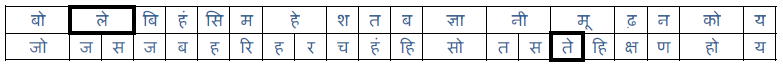Note that the maatraa of ले in ‘बोले’ in the first line is 2 whereas the maatraa of ते in तेहि is 1. This is apparent from their pronunciation. The letter ‘le’ in ‘bole’ is pronounced elongated whereas ‘te’ in ‘tehi’ is pronounced in short.

In the first line consider what happens if you replace ‘मूढ़’ with ‘मूर्ख’. It does not violate the maatraa structure or the meaning. Note, that half ‘r’ in moorkh does not contribute any maatraa because its pronunciation is telescoped in ‘moo’.

In general, the following logic determines when a half letter will consume 1 maatraa and when it will consume no maatraa:

• If a half letter is after a ‘laghu’ (लघु) swar of one maatraa, it would contribute a single maatraa. For example, र् in सुर्ख़ and सर्दी, ख् in सख्त consumes 1 maatraa. Thus सुर्ख़ and सख्त both consume 3 maatraas total and सर्दी consumes 4 maatraas total.
• If a half letter is after a deergh (दीर्घ) swar of 2 maatraas, it would not contribute any maatraa. For example, आत्म and आम both are 2+1 maatraas, i.e. the त् in आत्म does not contribute to the maatraa count as it is followed by the deergh आ.
• If a half letter is between two deergh swar, then the half-letter does consume one maatraa independently for itself. For example त् in आत्मा does consumes one maatraa and the total maatraa for this word is 2+1+2 = 5.

One way to verify these cases and similar queries that might arise regarding half letters is to say the word without the half letter and observe whether it impacts the time duration. Saying आमा (instead of आत्मा) does impact the time and a clear unit of time is missing. Saying दीघ (instead of दीर्घ) does not impact the time indicating that र् in दीर्घ is not consuming a maatraa.

Now let us take one more example, in which the rule of maatraa alignment is compromised, rather manipulated, by adding ‘lahraa’ or by elongating a letter or by giving a pause:

 कामिहि(2+1+1) नारि(2+1) पियारि(1+2+1) जिमि,(1+1) लोभिहि(2+1+1) प्रिय(1+1) जिमि(1+1) दाम(2+1) = 24 तिमि(1+1) रघुनाथ(1+1+2+1) निरंतर –अ -(1+1+1+1+1+1) प्रिय(1+1) लागहु(2+1+1) मोहि(1+1) राम(2+1) = 24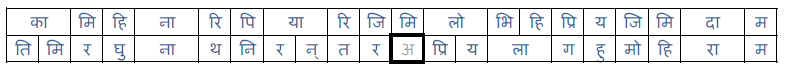The extra letter अ at the end of nirantar is a kind of lahra that elongates the last letter ‘र’. You can also pronounce it as ‘निरन्तरः’ or ‘निरन्तरा’ to get the same effect.

Finally, in this context, notice that the total maatraa of a line of doha is 24 in contrast to 16 of a chaupaayee. The series of maatraa is also different. A doha in general ends with a single maatraa and would end as 2+1 or 1+1+1. This gives the characteristic change of meter between a doha and a chaupaayee. This is true for any doha, as for example, written by Rahim.

रहिमन वे नर मर चुके, जे कहुं मांगन जाहिं
उनते पहिले वे मुये, जिन मुख निकसत नाहिं.

As a self-exercise, you may like to out the maatraa structure of this doha and verify that it has the same structure as the two dohas quoted above. Pick up some other dohas and do the same exercise.

Now consider the maatraa structure of Jhansi Kee Rani. Consider the same piece as quoted in Part 1:

 बुंदेले(1+1+2+2) हरबोलों(1+1+2+2) के(2) मुँह(1+1) हमने(1+1+2) सुनी(1+2) कहानी(1+2+2) थी,(2) = 30 खूब(2+1) लड़ी(1+2) मर्दानी(1+1+2+2) वह(1+1) तो(2) झाँसी(2+2) वाली(2+2) रानी(2+2) थी(2) = 30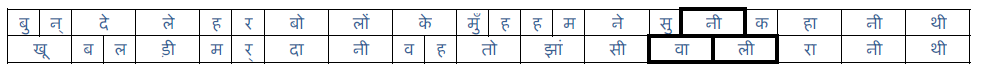Note that the total maatraas are 30 and the series matches at the beginning and the end of each line. Even though सुनी and वाली do not align, it does not hamper the rhythm because it is in the middle of the lines.

Now consider this antara
 जाओ(2+2) रानी(2+2) याद(2+1) रखेंगे(1+2+2) हम(1+1) कृतज्ञ(1+1+1+1) भारतवासी,(2+1+1+2+2) = 30 यह(1+1) तेरा(2+2) बलिदान(1+1+2+1) जगावेगा(1+2+2+2) स्वतंत्रता(1+1+1+1+2) अविनाशी,(1+1+12+2) = 30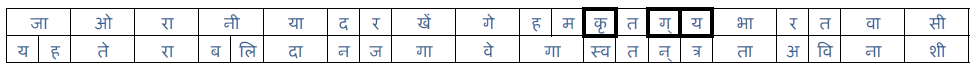Again note how the total maatraas =30 and the series of maatraas match. In counting the maatraas, note that kri (कृ) in the first line is one maatraa and gya (ज्ञ) is counted as 1+1 because of their pronunciations in the respective words. If a word starts with ज्ञ, in general it would be one maatraa because it is a composite of half ग and full य with half ग telescoped into य.

The main thing to note is that the total number of maatraas of the lines in sthaayee, sahyogee, and the antaraa match. This ensures the rhythm of the poem. You can verify it for other lines in the antara.

4. Example- Urdu ghazal
Now let us also consider a Urdu ghazal. The definition and the structure of the ghazal will be discussed in Part 4. For the present purpose of maatraa count, only the basic features of a ghazal are given.

Urdu ghazal has special rules. It consists of couplets (sher शेर). In the first couplet called matlaa, both lines must rhyme and have exactly the same maatraa structure (same total as well as same order). In the subsequent couplets, the second line must rhyme with both the lines of the matlaa and must have the same maatraa structure as both the lines of the matlaa. The first line of each subsequent couplet should not rhyme with the matlaa but that is not a strict rule. The maatraa structure of the first lines of each sher must be the same as each other and, in general, match with matlaa. The last couplet should have the poet's name though some poets (very few, notably Sahir) have not followed this tradition. In fact Sahir did not write ghazals anyway (very few, mainly in movies).

Now let us see the above rules illustrated with a familiar ghazal from Shakeel. This ghazal has been beautifully sung by Beghum Akhtar. The maatraa count of this ghazal is given below:

 बेज़ुबानी(2+1+2+2) जुबां(1+2) न(1) हो(2) जाये(2+2) = 17 राज़े-उलफ़त(2+1+1+1+1+1) अयाँ(1+2) न(1) हो(2) जाये(2+2) = 17

(Ayaan means to expose)

This is matlaa. Notice both the lines rhyme as 'jaaye'. The number of maatraas in each line is 17. The maatraa structure is same- if there is 2 in the top line, the bottom line at that place is either 2 or 1+1. Notice that ए in बेज़ुबानी is counted as 2, whereas in राज़े it is counted as 1 because it has a long pronunciation in bezubaanee and a short pronounciation in raaze. In general in composite possessive nouns in urdu, ए has a short pronunciation and has 1 maatraa (For example - गमे-दिल). Here is another couplet in the same ghazal.

 ज़िक्र(1+1+1) उनका(1+1+2) जुबान(1+2+1) पर(1+1) आया(2+2) = 17 यह(1+1) कहीं(1+2) दास्ताँ(2+1+2) न(1) हो(2) जाये(2+2) = 17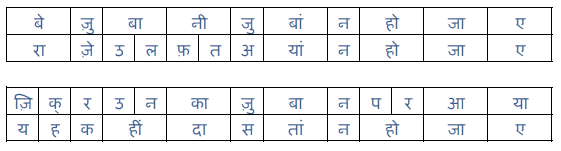Notice that the number of maatraa in each line is 17 and each line has the same maatraa structure as the matlaa. The first line does not rhyme with the matla but the second line rhymes with jaaye.

Next: Software for Maatraa Counting

8th May 2013इस महीने :
'किसके संग गाए थे'
मिलाप दूगड़

रात यदि श्याम नहीं आए थे
मैंने इतने गीत सुहाने किसके संग गाए थे?

गूँज रहा अब भी वंशी स्वर,
मुख-सम्मुख उड़ता पीताम्बर।
किसने फिर ये रास मनोहर
वन में रचवाये थे?

शंका क्यों रहने दें मन में
चल कर सखि देखें मधुवन में
..

पूरी प्रस्तुति यहाँ पढें और सुनें...
वो खुशी जो कहीं नहीं हासिल।
जो मुअस्सर* नहीं ज़माने में।
ख़्वाबगाहों* से चल के आएगी
ख़ुदबख़ुद तेरे आशियाने में।

~ विनोद तिवारी

*मुअस्सर: प्राप्त करने योग्य; ख्वाबगाहों - सपनों की जगह;

संकलन "समर्पित सत्य समर्पित स्वप्न" में कविताओं के बीच बीच कई मुक्तक भी हैं, जैसे कि यह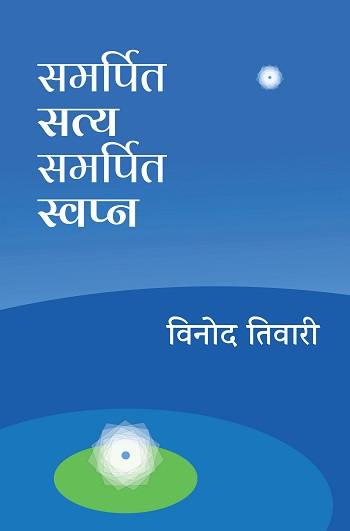तोड़ दो सीमा क्षितिज की,
गगन का विस्तार ले लो

विनोद तिवारी की कविता "प्यार का उपहार" का वीडियो। उपहार उनका और वीडियो द्वारा उपहार का सम्प्रेषण भी वह ही कर रहे हैं। सरल श्रृंगार रस और अभिसार में भीगा, फिर भी प्यार का उपहार ऐसा जो व्यापक होने को प्रेरित करे।

प्यार का उपहार